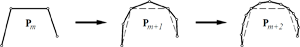# Using the Discrete Fourier-Transform to Analyze the Convergence of Subdivision Schemes

Leif Kobbelt
Applied and Computational Harmonic Analysis 5 (1998), Academic Press, pp. 68 - 91While the continuous Fourier transform is a well-established standard tool for the analysis of subdivision schemes, we present a new technique based on the discrete Fourier transform instead. We first prove a very general convergence criterion for arbitrary interpolatory schemes, i.e., for non-stationary, globally supported or even non-linear schemes. Then we use the discrete Fourier transform as an algebraic tool to transform subdivision schemes into a form suitable for the analysis. This allows us to formulate simple and numerically stable sufficient criteria for the convergence of subdivision schemes of very general type. We analyze some example schemes to illustrate the resulting easy-to-apply criteria which merely require to numerically estimate the maximum of a smooth function on a compact interval.